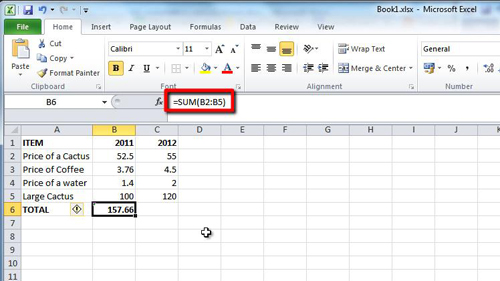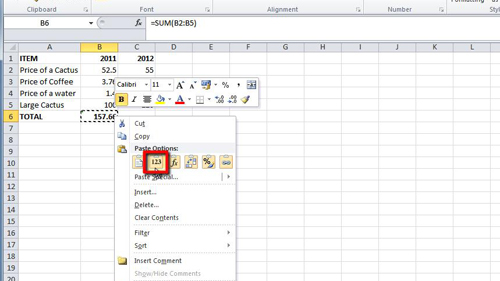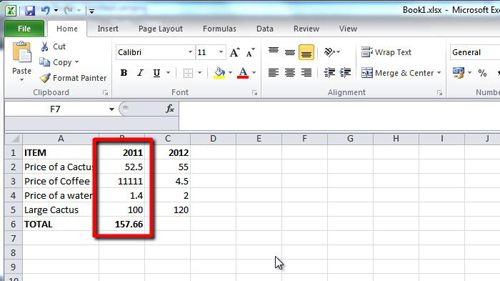## How to Make Excel 2010 formulas permanent

Once you have used an Excel formula to work something out it can be useful to keep this value by making it permanent. This tutorial shows you how to make excel formulas permanent.

Step # 1 – Using SUM to Create a Value

Start Excel and load a sheet with some information. Here we are going to use SUM to add the figures in the “2011” column. In cell “B6” type “=sum(B2:B5)” and press return. The result of the sum will appear in the cell. If you change one of the values in any of the selected cells the formula will change the result automatically, this is what we want to stop.Step # 2 – Making the Value Permanent

To learn how to make excel formulas permanent you need to remove the formula but keep the value. Click on the cell and click “Copy” in the top left of the window. Now right click on the same cell, “B6” here, and in the menu that appears locate the “Paste Values” icon below the “Paste Options” title. This will only paste the value and leave behind the formula.Step # 3 – Checking the Permanence of the Value

Now when we change the values in the cells above the formula the value that the formula calculated will not change. This means the formula has been given a permanent value.